Diameter

If the endpoints of a diameter of a circle are A(10, -1) and
B (3, 10), what is the radius of the circle?

Result

r =  6.5

Solution:Leave us a comment of this math problem and its solution (i.e. if it is still somewhat unclear...):Be the first to comment!To solve this verbal math problem are needed these knowledge from mathematics:

For Basic calculations in analytic geometry is helpful line slope calculator. From coordinates of two points in the plane it calculate slope, normal and parametric line equation(s), slope, directional angle, direction vector, the length of segment, intersections the coordinate axes etc. Pythagorean theorem is the base for the right triangle calculator.

Next similar examples:

1. Tree trunk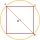What is the smallest diameter of a tree trunk that we can cut a square-section square with a side length of 20 cm?
2. Round table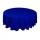Round table with diameter d = 105 cm is coated by square tablecloth with a side length 121 cm. About how many cm is higher center of tablecloth than its cornes?
3. Equation of circlefind an equation of the circle with indicated properties: a. center at (-3,5), diameter 20. b. center at origin and diameter 16.
4. Chord 3What is the radius of the circle where the chord is 2/3 of the radius from the center and has a length of 10 cm?
5. Seagull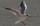Artificially created island in the shape of a circle with a radius of 50 m is overgrown with grass. The only exception is a landing area for helicopters in the shape of a rectangle measuring 15 m and 8 m. What is the probability that the flying seagull (wi
6. Circle's chordsIn the circle there are two chord length 30 and 34 cm. The shorter one is from the center twice than longer chord. Determine the radius of the circle.
7. Chord distanceThe circle k (S, 6 cm), calculate the chord distance from the center circle S when the length of the chord is t = 10 cm.
8. Chord 5It is given circle k / S; 5 cm /. Its chord MN is 3 cm away from the center of the circle . Calculate its length.
9. Chord circleThe circle to the (S, r = 8 cm) are different points A, B connected segment /AB/ = 12 cm. AB mark the middle of S'. Calculate |SS'|. Make the sketch.
10. The chordCalculate a chord length which the distance from the center of the circle (S, 6 cm) equals 3 cm.
11. SatinSanusha buys a piece of satin 2.4 m wide. The diagonal length of the fabric is 4m. What is the length of the piece of satin?
12. The cellar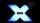Mr Novák has a cellar and a cellar window in the chalet has 0.6 meter square window. The window wishes to place an X-shaped grid in a square. He uses iron welded bars. Calculate the lengths of individual bars and what the total length of the bars he has t
13. Theorem proveWe want to prove the sentence: If the natural number n is divisible by six, then n is divisible by three. From what assumption we started?
14. Midpoint of segmentPoint A has coordinates [-16; 23] and the midpoint of the segment AB is the point [2; 12]. What are the coordinates of point B?
15. Diamond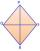Calculate the length of the two diagonals of the diamond if: a = 13 cm v = 12 cm
16. DistanceWha is the distance between the origin and the point (18; 22)?
17. UmbrellaCan umbrella 75 cm long fit into a box of fruit? The box has dimensions of 390 mm and 510 mm.